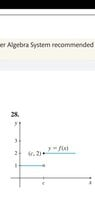# Investigate Limit of Piecewise Function

• nycmathguy

#### nycmathguy

Homework Statement
Graphs & Limits
Relevant Equations
Piecewise Function
My apologies. I posted the correct problem with the wrong set of instructions. It it a typo at my end. Here is the correct set of instructions for 28:

Use the graph to investigate limit of f(x) as x→c. If the limit does not exist, explain why.

For (a), the limit is 1.

For (b), the limit DOES NOT EXIST.

You say?

For (c), the LHL does not = RHL.

I conclude the limit DOES NOT EXIST.

#### Attachments

•Screenshot_20210613-150914_Drive.jpg
8.1 KB · Views: 71
Last edited by a moderator:
For (a), the limit is 1.
How is that possible? 1 is not in the domain of the function as x -> 2, just when you GET TO 2.

I think the problem here is that you are using the concept of "limit" when it should not be applied. The VALUE of the function is 2 when x=2. Period.

How is that possible? 1 is not in the domain of the function as x -> 2, just when you GET TO 2.

I think the problem here is that you are using the concept of "limit" when it should not be applied. The VALUE of the function is 2 when x=2. Period.
Yes, but ##\displaystyle{\lim_{x \nearrow c}f(x)=1}##. ##f(2)## cannot be determined.

Homework Statement:: Graphs & Limits
Relevant Equations:: Piecewise Function

Use the graph to investigate the following:

(a) lim of f(x) as x→2 from the left side.

(b) lim of f(x) as x→2 from the right side.

(c) lim of f(x) as x→2.

For (a), the limit is 1.

For (b), the limit is 2.

For (c), the LHL does not = RHL.
You should say ##x \to c## not ##x \to 2##. The last one means, that the ##f(x)## is not continuous at ##x=c##. ##f(c)=2## but ##\lim_{x\to c} f(x)## has no determined value, because both possible paths lead to a different value.
I conclude the limit for y = f(x) DNE.
?

Yes, but ##\displaystyle{\lim_{x \nearrow c}f(x)=1}##. ##f(2)## cannot be determined.
We are interpreting the graph in opposite ways. I interpret the black to dot to be a value and the white dot to be empty and you are interpreting it the opposite way. Is there are standard for this?

EDIT: your follow-on post clarifies this

We are interpreting the graph in opposite ways. I interpret the black to dot to be a value and the white dot to be empty and you are interpreting it the opposite way. Is there are standard for this?
Me, too. But you supposed ##c=2## which hasn't been said.

Me, too. But you supposed ##c=2## which hasn't been said.
see my EDIT

How is that possible? 1 is not in the domain of the function as x -> 2, just when you GET TO 2.

I think the problem here is that you are using the concept of "limit" when it should not be applied. The VALUE of the function is 2 when x=2. Period.

See my editing work.

Yes, but ##\displaystyle{\lim_{x \nearrow c}f(x)=1}##. ##f(2)## cannot be determined.

See my editing work. If f(2) cannot be determined due to the hole at (1, 2), the LHL DOES NOT = RHL. I conclude the limit does not exist.

You say?

See my editing work. If f(2) cannot be determined due to the hole at (1, 2), the LHL DOES NOT = RHL. I conclude the limit does not exist.

You say?
The (two-sided) limit cannot not detemined, ##f(c)=2## can, as can the one-sided limits.

Me, too. But you supposed ##c=2## which hasn't been said.

My apologies. I posted the correct problem with the wrong set of instructions. It it a typo at my end. Here is the correct set of instructions:

Use the graph to investigate limit of f(x) as
x→c. If the limit does not exist, explain why.

see my EDIT

My apologies. I posted the correct problem with the wrong set of instructions. It it a typo at my end. Here is the correct set of instructions:

Use the graph to investigate limit of f(x) as
x→c. If the limit does not exist, explain why.